# How to put values in associative array into another array PHP

Last Updated on

CraftyTechie is reader-supported. When you buy through links on our site, we may earn an affiliate commission.

Readers interested in the tutorials and examples can skip directly to the options and examples of how to put values in associative array into another array PHP. However, reading through the essential concepts is highly recommended to get more clarity on the subject.

## Intro

We have seen various arrays operations over time in different articles. All these are greatly helpful in development and programming. A firm grip on these concepts will help you a long way, as arrays are a crucial data structure and lay a cornerstone for implementing other abstract data types like linked lists, stacks, or queues.

On the same note, we will see another array operation, and that is to put values in associative array into another array PHP. In simpler terms, it could be copying an associative array. We need to get familiar with two important programming terms before diving into the main subject.

These two crucial concepts are related to how a programming language stores a variable. Typically, there are two ways of storing a variable. One way is to store references and another is to store values. We distinguish such variables by value type and reference type.

## Value Type

A value type variable stores its content in a memory independent of other variables. Whenever we assign a value to a value type variable, the system allocates separate memory to it.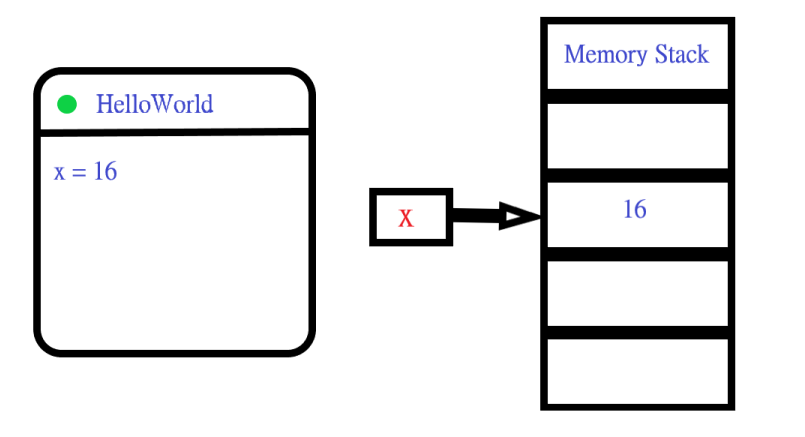The figure tries to illustrate a program that assigns a variable `x` a value of `16`. On the right, a memory stack allots a memory slot to the variable. Now, the following figure clarifies the most crucial aspect of the value type variables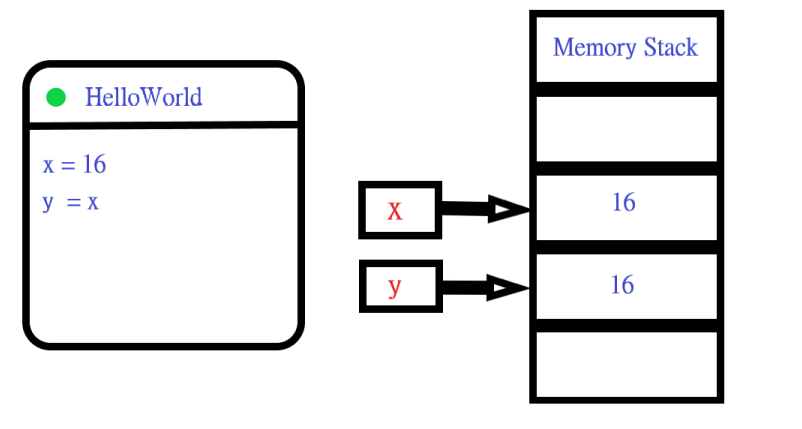The program declares a variable `y` and assigns it to variable` x`. As discussed, variable `y` is a value type variable and thus gets a separate memory slot. It gets a value of `16` because we’ve assigned it to `x`

What if we change the value of the variable `x`? Would it affect the value of `y`? Give it a good thought before viewing the following figure.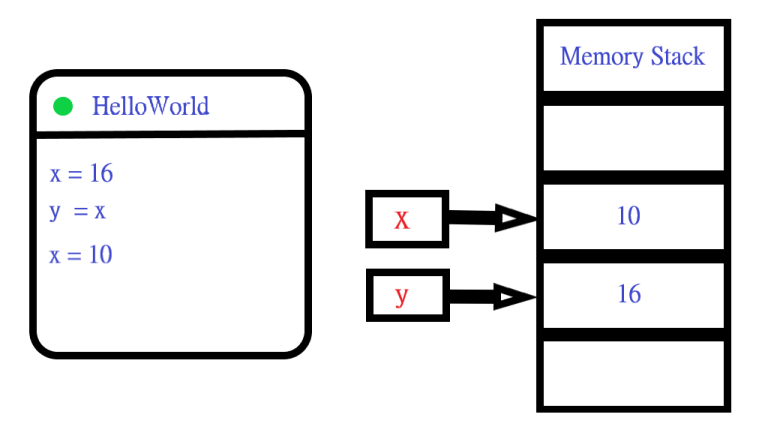Changing variable `x` doesn’t affect variable `y`. This fact is also true for the vice versa. The reason is that both these variables are value types.

There is another term known as deep copy. You’ve already seen what deep copy is. When you copy one variable’s value to another, the variables store these values independently in a separate memory slot.

The opposite of a deep copy is shallow copy. The idea of shallow copy will be clear after we understand reference type variables which is the next thing in line.

## Reference Type

Reference type variables store a reference or pointer to another memory slot. At first, this would sound confusing. Think of it this way, a variable stores a value, but it is an address or reference to another memory slot.

So when you try to access a reference type variable, it goes to the memory and grabs that address. This address leads to a memory slot that could contain a value, say an array. Don’t worry!! The following figure clarifies it further.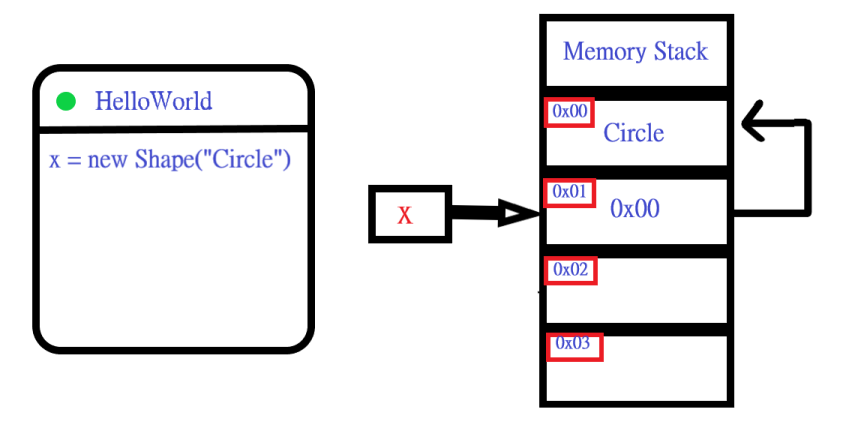We changed the figure, and it now includes the memory addresses of each slot, as shown in the red-bordered boxes. These addresses serve the same purpose as home addresses, but we have neighboring memory slots instead of homes here.

The variable `x` in the program is an object, and objects are reference type variables. Basically, `x` stores a reference to `0x00`. It is an address to the first memory slot. The first memory slot has a shape object which is a circle.

A common misconception arises when programmers say `x` is an object instead of saying that it is a reference to an object. As we’ve mentioned that objects are reference types, that’s why it is always implicitly intended that the variable is a reference to an object that is residing somewhere in the memory.

Now, consider the following figure. See what happens when we declare a variable `y` to assign it to `x`.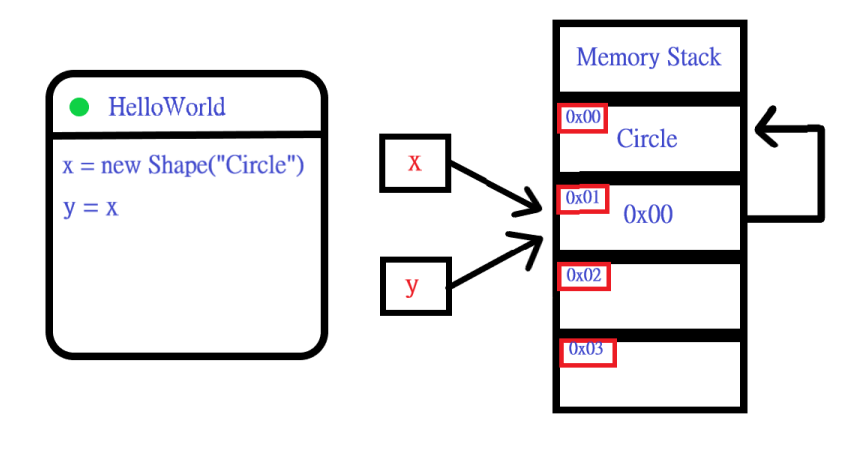Both `x` and `y` refer to the same memory address now. It implies that `x` and `y` have access to the exact memory location and thus the same circle object. Can you anticipate what happens if we reassign a new shape to `y`?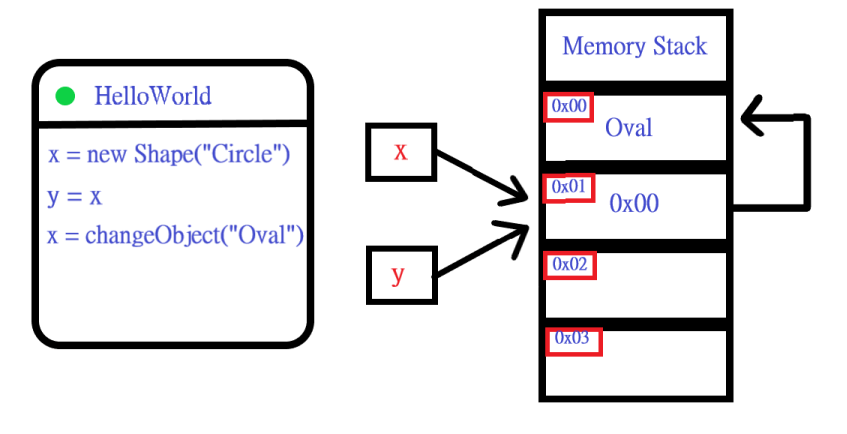Modifying the variable `y` changes the object to oval. This change will be reflected for both the variables because they both still refer to the same object. Remember, we’ve seen the term shallow copy. A shallow copy is copying a reference instead of value. It is similar to what we’ve seen while assigning `x` to `y`.

## Arrays: Are they Value Type or Reference Type?

Arrays are reference types in Javascript, Java, Python, and many other programming languages. However, arrays are value types in PHP. That’s why in PHP, we can directly assign an array to another variable, and it would always be a deep copy. This direct assignment option is the next thing we’re going to explore.

## Option#1 – Copy array PHP using direct assignment

Arrays are value types in PHP, and thus we can simply assign it from one variable to another. Let’s look at an example.

``````<?php
#An associative array.
\$array_1 = array("Number"=>1, "String" => "Hello World", "Number_Array"=>[1,2,3],"Boolean"=>"True");

#Assigning \$array_1 to \$array_2.
\$array_2 = \$array_1;

/*
OUTPUT
Array
(
[Number] => 1
[String] => Hello World
[Number_Array] => Array
(
 => 1
 => 2
 => 3
)

[Boolean] => True
)
*/
print_r(\$array_2);

//Change String to New World.
\$array_2["String"] = "New World";

/*
OUTPUT
Array
(
[Number] => 1
[String] => New World
[Number_Array] => Array
(
 => 1
 => 2
 => 3
)

[Boolean] => True
)
*/
print_r(\$array_2);

/*
OUTPUT
Array
(
[Number] => 1
[String] => Hello World
[Number_Array] => Array
(
 => 1
 => 2
 => 3
)

[Boolean] => True
)
*/
print_r(\$array_1);

?>
``````

We have an \$array_1 and we assign it to \$array_2. The \$array_2 is a deep copy, and changing it doesn’t affect \$array_1. Copying an array is convenient in PHP.

## Option#2 – Copy array PHP using a foreach loop

Using a foreach loop could be an option if you’re trying to filter out some keys or values based on their type and content. The following example copies values of types other than an array.

``````<?php
#An associative array
\$array_1 = array("Number"=>1, "String" => "Hello World", "Number_Array"=>[1,2,3],"Boolean"=>"True");

//Initially an empty array
\$array_2 = array();

//Iterate over \$array_1
foreach(\$array_1 as \$key=>\$value)
{
if(gettype(\$value) !== "array")
{
\$array_2[\$key] = \$value;
}
}

print_r(\$array_2);

/*
OUTPUT
Array
(
[Number] => 1
[String] => Hello World
[Boolean] => True
)
*/
?>
``````

A loop will give you more control of how you put values in associative array into another array PHP.

## Option#3 – Copy array PHP using array_merge

PHP array_merge function is ideal for copying more than one array into another in PHP. Let’s see how that works.

``````<?php
\$array_1 = array(
0 => "Bob",
1 => "Stacie",
2 => "Robert"
);

\$array_2 = array(
0 => "Anna",
1 => "Matthew",
2 => "John"
);

//Put the values of two arrays in \$employees_array
\$employees_array = array_merge(\$array_1,\$array_2);

print_r(\$employees_array);

/*
OUTPUT
Array
(
 => Bob
 => Stacie
 => Robert
 => Anna
 => Matthew
 => John
)
*/
?>
``````

Great!! PHP array_merge puts the values of two arrays into another array. Plus, it also takes care of reindexing.

## Conclusion

That’s pretty much all about this article. We’ve discussed some crucial concepts that could help you in the long run. They are frequently asked questions in job interviews and assessments. They are central to understanding various algorithms, data structures, and programming in general. Finally, we explored different options of how to put values in associative array into another array PHP.

## Want to explore further about PHP arrays?

We have many fun articles related to PHP arrays. You can explore these to learn more about arrays in PHP.

How to tell if an element is present in an associative array PHP

Remove element from an associative array in PHP

How to split associative arrays in PHP